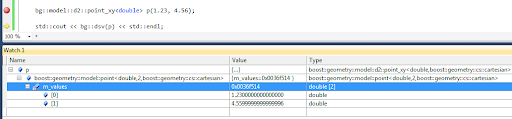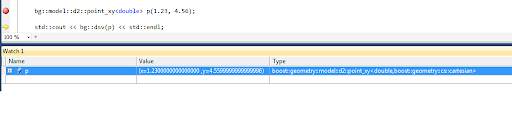# Debugger Visualizers

When debugging in Visual Studio, watching the contents of a variable can be of great help. But as soon as a variable is of a custom type, it might be inconvenient.

For example Boost.Geometry. You can examine the contents of a point or a polygon in the Debugger Watch Window. But the representation of a point type is cumbersome: "{...}". If you click that open, you will see m_values and a meaningless pointer. Still awkward. If you click that m_values open, you see it again, in the Values column the same pointer again. Still no coordinates. If you click that pointer open, yes, you finally see the values! But it takes three steps (clicks).If you examine a polygon, you have to do these three steps for each point in each of its rings!

You will be tired of that soon, especially if you know it can be helped using the Visual Studio Debugger Visualizers. It seems this method is not documented by Microsoft, so it is a bit of hacking, but descriptions can be found on the web (references below).

This is the recipe how to do it:
• go to the folder "c:\Program Files (x86)\Microsoft Visual Studio 10.0\Common7\Packages\Debugger" (usually there, path may change, this path is for VS 2010)
• make a backup of the file autoexp.dat because we are going to change it
• open autoexp.dat in an editor (it is (on Windows 7) convenient to edit in another path, because saving requires Administrator rights)
• go to the bottom, just before [hresult]
• enter the cryptic lines shown below this bullet list
• save the file (if you did edit it in another path, copy it back, giving Administrator rights)
These are the lines to add:
`boost::geometry::model::d2::point_xy<*,*>{   preview ( #("(x=", \$e.m_values, " ,y=", \$e.m_values, ")") )}`
It is a bit abstruse. In preview ( #( ) ) you have to enter member variables of your class (which should be called \$e for enigmatic reasons). You can mix them up with strings. All is comma separated. Note that the parentheses should be placed as displayed.

After this exercise, restart Visual Studio and debug again and... you will see a much better appearance of our point in the Watch window:We don't have to click at all. Our point is just there, x- and y- coordinates specified. Oh yes, 4.56 is now 4.55999 but that is something different, it has to do with floating point precision. We ignore that in this blog.

## Definition for Boost.Geometry

At this moment I have point_xy but also model::point defined. I could have more but this is already very useful. Linestrings or polygons automatically use these point presentations, and because std::vectors are visualized by Microsoft (in that same file autoexp.dat), it is all very clear in the Watch Window.

So the section I defined looks like this:

`; BOOST.GEOMETRYboost::geometry::model::point<*,2,*>{   preview ( #("(", \$e.m_values, " , ", \$e.m_values, ")") )}boost::geometry::model::point<*,3,*>{   preview ( #("(", \$e.m_values, " , ", \$e.m_values, " , ", \$e.m_values, ")") )}boost::geometry::model::d2::point_xy<*,*>{   preview ( #("(x=", \$e.m_values, " ,y=", \$e.m_values, ")") )} `
I have it running in Visual Studio C++ 2010 Express, and also in Visual Studio C++ 2005 Express. Debugging visualizers is a bit tricky, if you make an error you might get an exception window. But in version 2010 it is better than it was in version 2005.

## References

There is more than this. More is explained on these pages: And in .NET (but this is actually another solution, implementing a custom visualizer in code):

# SqlGeometry and Boxes

I wanted to write a blog about partitioning (calling an algorithm using an on-the-fly quadtree or octree) and how it is implemented in Boost.Geometry, and how it could also be used / implemented for the SqlGeometry type. But somehow that blog will be too large and I blog here just about a box/rectangle in SqlGeometry. It is not too difficult, but I just googled and did not find other blogs saying the same things, so I hope it adds something.

## STEnvelope

The OGC/ISO STEnvelope function, supported by spatial databases and many geometry libraries, returns the envelope of a geometry. An envelope is: a bounding box or bounding rectangle, also known as an axis aligned bounding box (aabb), a bbox, a minimum bounding rectangle (mbr) or an extent.

More in detail, STEnvelope returns a Geometry object describing a rectangle. OGC does not know the notion of a box. That is sometimes a bit inconvenient, but it is how it is. Boost.Geometry created a Box Concept, because Boost.Geometry is strongly typed, and envelopes returning polygons with always four or five points would confuse the non-OGC users of our library.

I blogged earlier about the spatial extent of a query in SQL Server, here.

A box is a simple geometry, with (in 2D) a minimum x and y and a maximum x and y. But how to get that from a geometry in a spatial database... I mean, the envelope returns a polygon containing five points (assuming it is closed), is the first point lower left? And is that guaranteed? The OGC specifications say: The minimum bounding box for this Geometry, returned as a Geometry. The polygon is defined by the corner points of the bounding box [(MINX, MINY), (MAXX, MINY), (MAXX, MAXY), (MINX, MAXY), (MINX, MINY)]. So yes, it should be guaranteed.

Let's look at it in more detail.

## SQL Server

In SQL Server it indeed seems the case that the first point of the polygon returned by STEnvelope is the lower left point. Then, the third point must be the upper right point. Because, if you consider the polygon clockwise, it is the third point, and if it is counterclockwise, it is also the third point.

We use this query:

`select geometry::STGeomFromText('POLYGON((0 0,2 5,4 1,0 0))',0).STEnvelope().STPointN(3).STAsText()`

... to get the third point (which should be upper right), and it indeed gives me: POINT(4 5)

## PostGIS

In PostGIS, the first point of the polygon returned by ST_Envelope is the lower left point as well. Great.

As you (maybe) know, SQLGeometry uses methods, PostGIS uses functions. So the corresponding nested function calls would be:

`select ST_AsText(ST_PointN((ST_Envelope(ST_GeomFromText('POLYGON((0 0,2 5,4 1,0 0))',0))), 3))`

But... no result, we get a null value back. Looking at the OGC specs, this is correct: NumPoints is defined for a LineString and not for a Polygon. SqlServer is somewhat more relaxed here (as is Boost.Geometry), returning the total number of points of a polygon (including interior rings, if any).

OK, behaviour is correct, so we try again, now inserting the ST_Boundary function (making a linestring of the boundary of a polygon). Our new function call is:

`select ST_AsText(ST_PointN(ST_Boundary((ST_Envelope(ST_GeomFromText('POLYGON((0 0,2 5,4 1,0 0))',0)))), 3))`

... and we have our upper right point. Using a 1 for the last value (the index of ST_PointN is one-based) would return the lower left point.

## Boost.Geometry

Boost.Geometry, as said, returns a box (conforming a Box Concept) for the envelope (or assigns one). So this is more or less outside the discussion... Having a box you can get the minimum-corner coordinates (might be 2D, might be 3D, or more) and the maximum-corner coordinates.

## SqlGeometry

I blogged about the useful SqlGeometry type here. The SqlGeometry type is polymorphic, it can be any OGC geometry. But, therefore, it cannot be a Rectangle (because, remember, a rectangle is not an OGC Geometry). So if it contains a Rectangle, it is a Polygon.

Using C#, it would be useful to get the coordinates from a Polygon returned by STEnvelope and that goes like this. Note that (in the light of defensive programming) we don't even assume that the first point is the lower left point. We add this code as a function (we could add it to a class GeometryExtensions, but for now we don't). It is called BoxToCoordinates, see the code below.

And, for symmetry, and because it is convenient, or often necessary (last reason most important ;-) ) we add a counterpart function as well, which creates a rectangle for you given four coordinate values. We call that one CoordinatesToBox, see code below.

## The code

OK, this was some preparation for next blog, not too difficult, and now we can convert an STEnvelope result to coordinate values, and back. The full code is displayed below. Between BoxToCoordinates and CoordinatesToBox, there will be some more interesting calculations in the next blog. Stay tuned.

`using System;using Microsoft.SqlServer.Types;namespace BlogSqlGeometryBox{    class Program    {        public static SqlGeometry FromWkt(string text, int srid)        {           return SqlGeometry.STGeomFromText(new System.Data.SqlTypes.SqlChars(text), srid);        }        public static SqlGeometry CoordinatesToBox(double x1, double y1, double x2, double y2, int srid)        {           SqlGeometryBuilder builder = new SqlGeometryBuilder();           builder.SetSrid(srid);           builder.BeginGeometry(OpenGisGeometryType.Polygon);           builder.BeginFigure(x1, y1);           builder.AddLine(x1, y2);           builder.AddLine(x2, y2);           builder.AddLine(x2, y1);           builder.AddLine(x1, y1); // Yes, we close it neatly           builder.EndFigure();           builder.EndGeometry();           return builder.ConstructedGeometry;        }        private static void MakeFirstSmaller<T>(ref T a, ref T b) where T : IComparable        {           if (a.CompareTo(b) > 0)           {               // Exchange the two values               T t = a;               a = b;               b = t;           }        }        public static void BoxToCoordinates(SqlGeometry box, out double x1, out double y1, out double x2, out double y2)        {           SqlGeometry lower_left = box.STPointN(1);           SqlGeometry upper_right = box.STPointN(3);           x1 = lower_left.STX.Value;           y1 = lower_left.STY.Value;           x2 = upper_right.STX.Value;           y2 = upper_right.STY.Value;           // Defensive programming:           MakeFirstSmaller(ref x1, ref x2);           MakeFirstSmaller(ref y1, ref y2);        }        static void Main(string[] args)        {           SqlGeometry triangle = FromWkt("POLYGON((0 0,2 5,4 1,0 0))", 0);           SqlGeometry box = triangle.STEnvelope();           double x1, y1, x2, y2;           BoxToCoordinates(box, out x1, out y1, out x2, out y2);           // Do something with these coordinates. Let's create a larger box           x1 -= 1;           y1 -= 1;           x2 += 1;           y2 += 1;           box = CoordinatesToBox(x1, y1, x2, y2, 0);           System.Console.WriteLine(box.STAsText().ToSqlString().ToString());        }    }}`

Writing:

`POLYGON ((-1 -1, -1 6, 5 6, 5 -1, -1 -1))`